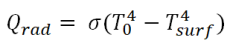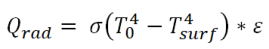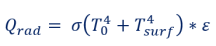## When the radiation model can be used?

If the radiation heat flow is comparable with other heat flow, which is the main heat flow source in the problem (for example, convective heat flow), you have to use a radiation model.

The radiation heat flow can be evaluated based on temperature difference between surrounding environment and the body’s surface using the Stefan-Boltzmann's law:The formula above represents the low for the absolutely black body (Plankian radiator). For real bodies you have to multiply the value by emissivity factor.## Simplified method of calculation the heat losses due to radiation

Examine the problem:

• Radiation extracts substantial amount of heat from a surface.
• The radiated heat doesn’t rereflects and doesn’t returns into the simulated system in substantial amount. This means that neither environment no adjacent surfaces are substantially heated because of radiation from the radiant surface.

You don’t have to use radiation model for solving such problems. You can just set a negative heat source on the surface. The appropriate heat flow can be calculated by formula:where epsilon is the emissivity factor of the surface, To is the temperature of the environment (it is taken as constant), Tsurf is the temperature of the surface.

So the larger is the difference between the temperatures of the surface and the environment, the larger is heat extraction.

But if the rejected heat is to be returned into the environment or on other surfaces, the proposed method is incorrect because it assumes heat extruction into infinity. Thus we have to use the radiation model.

The following radiation models are the most used:

• The diffusion radiation model uses the diffusion equation to calculate transfer of the radiation. So this model doesn’t take into account directions of the rays but calculations of the model are much quicker than calculations of ray tracing.
• The surface-to-surface model uses ray tracing from surface to surface, so it takes into account the directions of radiation and it can be used for simulation of radiation in closed systems. However, this model requires substantial computational resources.

Because of the nature of the radiation models, you should take into account their limited ranges of applicability. This must be considered when doing computational simulation. In paragraphs below we consider some ranges of applicability of the models, which are implemented in FlowVision.

## The models implemented in FlowVision

Currently (in version 3.10.01) FlowVision implements no surface-to-surface models. FlowVision 3.10.01 has models P1 and Optically thin layer models that are quick diffusion models.
Range of applicability of the model P1

The P1 model simulates radiation energy transfer as a diffusion process. This model assumes:

• local thermal equilibrium occurs in the medium
• the radiation field is isotropic (the radiation field is spatially uniform and there are no substantially expressed direction of radiation)
• scattering is isotropic

The model was named as P1 because it assumes that energy flux is proportional to the gradient of the radiation energy density; photons diffuse similarly to molecules in gas.

In translucent mediums and in situations, when the shape of the surface influences on the radiation field, this model can cause large inaccuracies.

The diffusion approach is usually applicable when the anisotropy of the radiation field is weak. This condition is fulfilled when the photon free path is much less thanthat the characteristic length of the problem.

However, the practice of solving various problems of radiation gas dynamics (RGD) shows that diffusion approximation often yields very good results.

An example of the problems, which are solved using this model, is simulation of radiation between very closely located surfaces.

## Range of applicability of the model of optically thin layer

This simplified model doesn’t require solving the equation for radiation density and specifying boundary conditions. The source term in the energy equation doesn’t take into account the radiation flux coming into the cell.

So the model actually takes into account radiation heat loss in each cell without transferring the heat to adjacent cells and on surfaces.

This model is only used in simulations of combustion to take into account radiation heat losses of the burning environment. It is assumed that heat doesn’t leave the computational area via radiation with no diffusion in translucent medium.